# Add Fractions

Several examples with detailed solutions and exercises with answers on addition of fractions, are presented.is used here to denote the fraction of a over b. a is called the numerator and b is called the denominator and must be non zero.

Examples of fractions: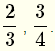NOTE:is undefined since division by zero is undefined in mathematics.

A calculator to add fractions is included in this website. You may use it to check your answers.

How to add fractions?

## 1. Add fractions with equal denominator

Example 1: Add and simplfy.Solution to Example 1

To add fractions with the same denominator, you add the numerators, keep the same denominator and simplify if possibleExample 2: Add, simplify and express the final answer as a fraction.Solution to Example 2

Add the numerators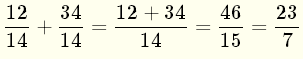## 2. Add fractions with non equal denominators

Example 3: Add, simplify and express the final answer as a fraction.Solution to Example 3

We first need to find the lowest common multiple (LCM) of the denominators 9 and 6 by factoring into prime factors.We next convert the two given fractions so that they have common denominator equal to the LCM = 18. The denominator of the fractionis 9 and we need to multiply numerator and denominator by 2 in order to change the denominator to 18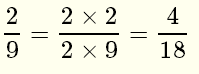The denominator of the fraction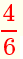is 6 and we need to multiply numerator and denominator by 3 in order to change the denominator to 18Now that we have converted the two fractions so that they have common denominator, we can easily add them as followsExample 4: Add, simplify and express the final answer as a fraction and as a mixed number.Solution to Example 4

Find the LCM of the denominators 15 and 55We convert the given fractions so the they have common denominator equal to the LCM = 165. The denominator of the fraction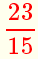is 15 and both numerator and denominator need to be multiplied by 11 in order to change the denominator to 165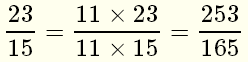The second fractionis converted to one with the denominator equal to 165 by multiplying numerator and denominator by 3 in order to change the denominator to 165We now add the fractions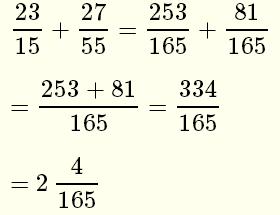Example 5: Add, simplify and express the final answer as a fraction.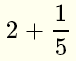Solution to Example 5

We first convert 2 into a fraction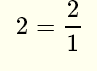The common denominator will be 5We now add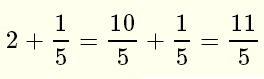Exercises: Add, simplify and express the answer as a fraction and as a mixed number.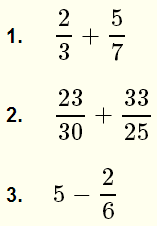Answers to Above Exercises:.More on fractions and fraction calculators.